The Theory \ Details \ portfolio optimization \ optimality criteria

# Portfolio Optimization

## Optimality criteria

The structure of investor’s optimal portfolio depends on objective factors (such as budget and administrative constraints on portfolio structure), as well as on the subjective preferences of the investor. The above preferences depend on both the investor’s attitude to risk and the character of the investor’s goals.

The formalization of investor’s preferences results in the formation of some optimality criterion.

The optimality criterion is any function dependent from portfolio weights. The structure of portfolio that is optimal relative to the selected criterion corresponds to the maximum (or minimum) value of such function (taking into account possible constraints).

Below are presented the optimality criteria that are contained in SmartFolio:

### CRRA Utility Function Criterion

Assume that utility function of the investor belongs to the CRRA class. Assume also that the relative risk aversion coefficient value is known and is equal to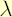. Consider the following functions:
1. Polynomial utility function2. Logarithmic utility function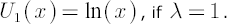The portfolio that is optimal relative to a CRRA utility function is obtained by the maximization (over the set of all admissible portfolios) of one of the above mentioned functions from the portfolio terminal wealth. According to the result stated above (which connects the Utility Function Approach and the Mean-Variance Approach) under the assumptions of the analytical model it is equivalent to the maximization of the quantity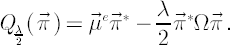### Analytically tractable optimal portfolios

Merton Portfolio
If the assumptions of the
analytical model hold and there are no constraints on portfolio structure, the portfolio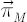that is optimal relative to a CRRA utility function allows for the analytical representation and is
referred to as Merton Portfolio.Let us consider some arbitrary portfolio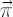. It might be informative to find out values of excess Mu that make the portfoliooptimal given the covariance matrix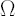and the relative risk aversion. From the above formula it follows that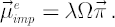Vector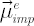, calculated above, is called Implied Excess Mu. If one wants to measure the distance between the existing and the optimal portfolios, one way to do it is to look at the difference betweenand the available estimate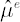of excess Mu.

Merton Portfolio with higher Interest Rate for Borrowing

The presented material is based on the results, obtained in
[Cvitanic, Karatzas; 1992]. Assume that analytical model holds, and the role of a riskless asset is played by cash. Consider situation, when the borrowing rate in cash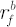is higher than the lending rate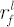.
Denote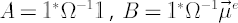, where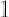is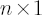vector of ones.denotes
relative risk aversion coefficient. Then weights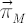of the Merton portfolio are calculated according to the following rule: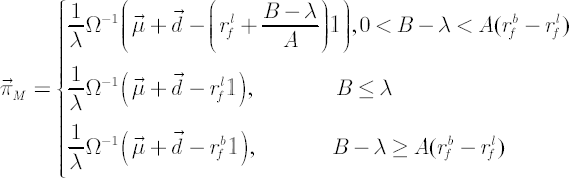Three-Fund Portfolio

The Three-Fund portfolio rule was proposed in
[Kan, Zhow; 2005] as an effective way to deal with model parameters uncertainty (it is assumed that the analytical model holds). Three-fund portfolio consists of three parts: riskless asset, tangency portfolio and global minimum variance portfolio. Corresponding weights are chosen to minimize the expected loss in utility due to the presence of an estimation error. In such case, the three-fund portfolio provides significant improvement in utility over the traditional Merton portfolio (which is effectively a two-fund portfolio), where the sample estimates are “plugged” in place of the population values. Three-fund portfolio rule has the following form: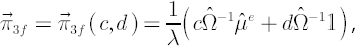wheredenotes
relative risk aversion coefficient;anddenote
sample excess Mu and sample covariance matrix respectively;is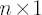vector of ones;are appropriate constants (see
[Kan, Zhow; (2005)] for exact formulas).

### Target Shortfall Probability Criterion

The criterion based on the maximization of CRRA of utility functions has one defect. The point is that the true value of the relative risk aversion coefficient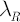is extremely difficult to estimate. Moreover, experiments exist that show thatof an investor can vary depending on specific circumstances. These reasons form the sufficient ground for the search of more direct optimality criteria. It seems that one of the most successful attempts in this direction was made by Michael Stutzer (see [Stutzer; 2003]).

The essence of Stutzer’s criterion is that an investor chooses the critical level for the excess growth rate he/she would like to beat in the future, whereupon minimizes the probability of falling short of the selected goal.

Note.
It is worth mentioning that Stutzer’s work develops ideas, first proposed by A. D. Roy in 1952 for the
single-period model. (see
[Roy; 1952])

Criterion algorithm
1. Investor selects his/her investment horizon.
2. Investor selects his/her target excess growth rate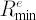over the risk-free rate.
Note. If investor’s goal is to beat by ratesome benchmark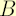, then portfolio wealth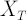must be denominated in units of. See
riskless asset.
3. Investor constructs the portfolio strategy with constant weightsthat maximize the probability of portfolio realized excess growth rate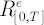on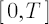exceeding.

Note. Portfolio strategy with constant portfolio weights is suboptimal in terms of minimizing the probability of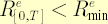event only. For example, if for some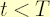portfolio wealth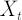exceeds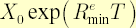, then target excess growth rateonis achieved with 100% probability by investing all funds for remaining time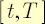to
riskless asset. To make optimal portfolio structure constant through time, target shortfall probability criterion should be defined a little bit more precisely.

Criterion definition. At every point in timeinvestor minimizes the probability of.

Optimal portfolio strategy, which corresponds to this more accurate definition, no longer depends on the current portfolio wealth and coincides with the portfolio strategyobtained in step 3.

Features of Optimal Portfolio• Optimal portfoliodoesn’t depend on.
• Volatility of optimal portfolio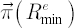increases with.

Calculation Techniques
Optimal Portfoliois obtained by solving the following problem:If the analytical model holds, then the stated problem is equivalent to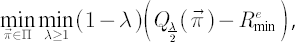wherecorresponds to risk-adjusted expected excess rate of return.

Note. Solutionto the above problem under the
analytical model assumptions can be obtained alternatively by maximizing the so-called information ratio, where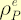is the portfolio expected excess growth rate. Such calculation technique is slightly more correct because it leads to the right answer even ifsurpasses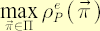. On the contrary, in the former case for all suchone obtains the same portfolio that maximizes the expected excess growth rate. However, the mentioned divergence is hardly significant in practice, since the choice of, surpassing, is almost always not economically justified: the resulted optimal portfolio corresponds to a value ofthat is strictly less than 1.
In the general case the information ratio may be replaced with
Sortino ratio or STARR ratio.

### Benchmark Tracking Criterion

As a rule, benchmark tracking criterion serves for the construction of portfolios that contain a modest number of assets and replicate dynamics of more diversified portfolio, such as market portfolio.

To apply benchmark tracking criterion two steps are required:
1. Select the benchmark asset and consider it further as a riskless asset.
2. Construct a fully-invested portfolio that minimizes some measure of Tracking Error between a portfolio and the benchmark subject to possible constraints.

In the analytical model framework the most appropriate measure of tracking error is portfolio volatility. Often it is coupled together with the lower constraint on portfolio expected excess growth rate.

In the general case of non-normal distribution of returns portfolio
downside volatility might appear a more plausible measure.

Minimum Acceptance Ratefrom the downside volatility definition may serve the same purpose as the lower constraint on portfolio expected excess growth rate.